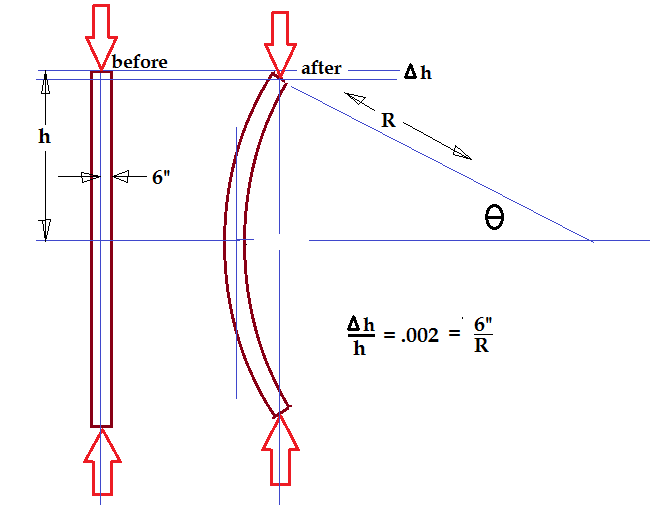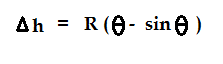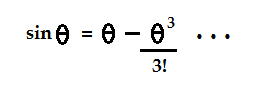## Saturday, June 9, 2012

### The Buckling Column: A Geometrical Approach

Today I'm going to post a calculation I did a long time ago when I was learning about structural theory. You probably know that a steel column, if sufficiently tall, will give out under load long before you reach the expected point of failure based on the elastic yield point. The column basically flips out on itself: it's called buckling, and it's the topic for today.

If a column is short enough, it won't buckle. It will just squish. The interesting mathematical question is: just how tall does a column have to be before it becomes prone to buckling? It's funny that this question can be answered by purely geometrical reasoning. You don't have to know the strength of steel or wood. Well, you have to know the ultimate deformation. For example, steel yields at a strain of close to 2000 parts per million. In other words, you can stretch or compress steel by two-tenths of one percent of its original dimension. Any column, even a short one, will buckle eventually if it doesn't otherwise fail. It's the onset of normal failure that marks the boundary between buckling and non-buckling columns.

My calculation is based on the small-angle formulas for a circular arc. There is one problem with this: at the point of buckling, a column will normally flip out into the shape of a sine wave. I'm going to ignore that detail and treat it as an arc of a circle circle. It means my answer won't be accurate, but it makes the math easier.

I'm going to take the column to be an I-beam, with a dimension of 12 inches flange-to-flange. Here is what my buckled column looks like:Why would the column choose to buckle instead of simply compressing? It will do so when it can achieve the same reduction in height with less energy. Instead of compressing uniformly, it will simply bend. If a column is too short, it will fail in simple compression before it has a chance to buckle. We are looking for the shortest column which will buckle before it fails. That is why the factor of 2000 ppm becomes critical, as I have shown in the diagram. It's interesting that this immediately gives us the radius of curvature at the moment of buckling...in this case, we get R = 3000.

You can imagine that just before it bends, the column has an epiphany. It asks itself: "why am I compressing both flanges when it would be just as easy for me to stretch one flange and compress the other?" It flips a coin and decides which way to bend: and once it bends, the centerline length returns to its normal, prestressed state! Now only the flanges are stressed. The left flange becomes longer, and the right flange becomes shorter. You can see in my drawing that the change in length in each flange is simply delta-h: it's the same change in length when you bend the column as when you compress the column...except when you compress it, both flanges get shorter, and when you bend it, one flange gets longer. Either way, it's the same compressional energy.

Using trig, it's straightforward to relate the change in length (delta-h) to the angle and the radius of curvature:Here's the part I like: we get to use the Taylor series for sin(theta):The cute thing about this is that we don't use the leading term, it's the third-order term that counts! Putting in the relation for delta-h and the value of R = 3000, I get....well, maybe I'll leave the math to you. There's some manipulation and eventually I get theta = 0.11, which eventually gives me h = 330.

Putting all the numbers together, I get potential buckling in a 12" I-beam when the height of the column is 660 inches or greater...a slenderness ratio of 55:1. I think the books might give a slightly different ratio but that's because I approximated the curvature of the buckled column as a portion of a circular arc instead of a sine wave.